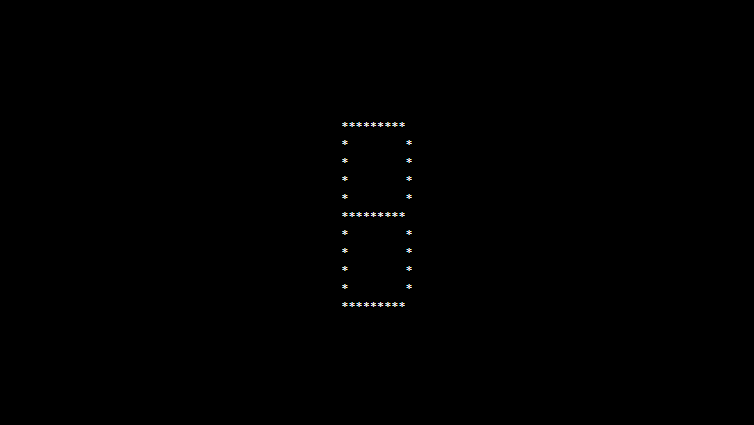# PHP program to print alphabet pattern B

In this article write a PHP program to print alphabet pattern B. This Program first takes the numbers of rows and then prints pattern using nested for loops.

## PHP program to print alphabet pattern B

```<?php
echo "<pre>";
for (\$row = 0; \$row < 11; \$row++) {
for (\$col = 0; \$col <= 11; \$col++) {
if (\$col == 1 or ((\$row == 0 or \$row == 5 or \$row == 10) and (\$col < 10 and \$col > 1)) or (\$col == 10 and (\$row != 0 and \$row != 5 and \$row != 10))) {
echo "*";
} else {
echo "&nbsp;";
}
}
echo "<br>";
}
echo "</pre>";
?>
```

### Output: